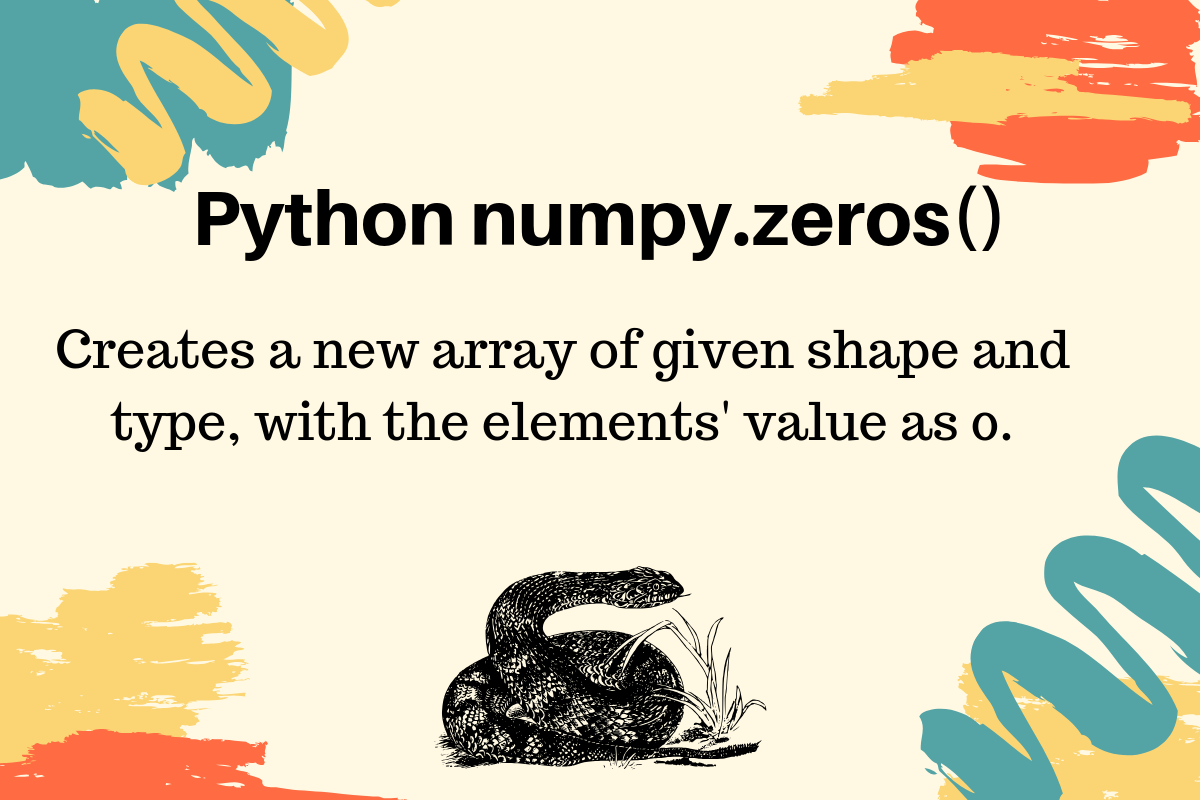# numpy.zeros() in Python

Published on August 3, 2022By PankajWhile we believe that this content benefits our community, we have not yet thoroughly reviewed it. If you have any suggestions for improvements, please let us know by clicking the “report an issue“ button at the bottom of the tutorial.

Python numpy.zeros() function returns a new array of given shape and type, where the element’s value as 0.

## numpy.zeros() function arguments

The numpy.zeros() function syntax is:

``````zeros(shape, dtype=None, order='C')
``````
• The shape is an int or tuple of ints to define the size of the array.
• The dtype is an optional parameter with default value as float. It’s used to specify the data type of the array, for example, int.
• The order defines the whether to store multi-dimensional array in row-major (C-style) or column-major (Fortran-style) order in memory.

## Python numpy.zeros() Examples

Let’s look at some examples of creating arrays using the numpy zeros() function.

### 1. Creating one-dimensional array with zeros

``````import numpy as np

array_1d = np.zeros(3)
print(array_1d)
``````

Output:

``````[0. 0. 0.]
``````

Notice that the elements are having the default data type as the float. That’s why the zeros are 0.

### 2. Creating Multi-dimensional array

``````import numpy as np

array_2d = np.zeros((2, 3))
print(array_2d)
``````

Output:

``````[[0. 0. 0.]
[0. 0. 0.]]
``````

### 3. NumPy zeros array with int data type

``````import numpy as np

array_2d_int = np.zeros((2, 3), dtype=int)
print(array_2d_int)
``````

Output:

``````[[0 0 0]
[0 0 0]]
``````

### 4. NumPy Array with Tuple Data Type and Zeroes

We can specify the array elements as a tuple and specify their data types too.

``````import numpy as np

array_mix_type = np.zeros((2, 2), dtype=[('x', 'int'), ('y', 'float')])
print(array_mix_type)
print(array_mix_type.dtype)
``````

Output:

``````[[(0, 0.) (0, 0.)]
[(0, 0.) (0, 0.)]]
[('x', '<i8'), ('y', '<f8')]
``````

Reference: API Doc

Thanks for learning with the DigitalOcean Community. Check out our offerings for compute, storage, networking, and managed databases.Pankaj

author

#### Still looking for an answer?

Ask a questionSearch for more help

JournalDev
DigitalOcean EmployeeJanuary 27, 2022

what is: <i <f ?

- James HH Rodriguez

JournalDev
DigitalOcean EmployeeApril 18, 2020

what does x=np.zeroes(W.shapre) do?

- het gala